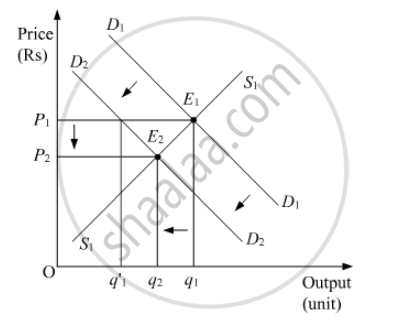# How Does the Equilibrium Price of a ‘Normal’ Commodity Change When Income of Its Buyers Falls? Explain the Chain Effects. - Economics

#### Question

How does the equilibrium price of a ‘normal’ commodity change when income of its buyers falls? Explain the chain effects.

#### Solution

Normal goods refer to those goods that share positive relationship with the income of the consumers. That is, when the consumers’ income rise (or fall), then the demand for the normal goods also rises (or falls). The effect of change in the consumers’ income on the demand of the normal goods is explained below diagrammatically.In the diagram, D1D1 represents initial demand curve and S1S1 represents initial supply curve. E1 is the initial equilibrium, where D1D1and S1Scurves intersect. OP1 is the initial equilibrium price and Oq1 is the initial equilibrium quantity.

Now, with a fall in the consumers’ income, the market demand for the normal goods fall. This is shown by the leftwards parallel shift of the curve to D2D2. At the initial price OP1, there exist excess supply equivalent tounits (i.e.). Due to the excess supply, competition among the producers increases as they try to get rid of the excess stock by selling their output at lower prices. The price will continue to fall until it reaches OP2,where, the new equilibrium is attained at point E2. At this new equilibrium, the new demand curve D2Dintersects the initial market supply curve S1S1. A comparison of the final equilibrium with the initial equilibrium indicates that due to the fall in the consumers’ income, both the equilibrium price as well as the equilibrium quantity has fallen.

Conclusion

Fall in Income ⇒ Decrease in Demand ⇒ Excess Supply at the existing price ⇒ Competition among the Producers Increases ⇒ Price Falls ⇒ New Equilibrium is attained ⇒  Reduced Equilibrium Quantity Demanded and Equilibrium Price

Is there an error in this question or solution?